Courses

# Test: Thin & Thick Cylinders - 2

## 10 Questions MCQ Test Topicwise Question Bank for Civil Engineering | Test: Thin & Thick Cylinders - 2

Description
This mock test of Test: Thin & Thick Cylinders - 2 for Civil Engineering (CE) helps you for every Civil Engineering (CE) entrance exam. This contains 10 Multiple Choice Questions for Civil Engineering (CE) Test: Thin & Thick Cylinders - 2 (mcq) to study with solutions a complete question bank. The solved questions answers in this Test: Thin & Thick Cylinders - 2 quiz give you a good mix of easy questions and tough questions. Civil Engineering (CE) students definitely take this Test: Thin & Thick Cylinders - 2 exercise for a better result in the exam. You can find other Test: Thin & Thick Cylinders - 2 extra questions, long questions & short questions for Civil Engineering (CE) on EduRev as well by searching above.
QUESTION: 1

### A thin cylindrical steel pressure vessel of diameter 6 cm and wall thickness 3 mm is subjected to an internal fluid pressure of intensity ’ p'. If the ultimate strength of steel is 3600 kg/cm2, the bursting pressure will be

Solution:

Hoop stress is more than longitudinal stress.
Hoop stress = pd/2t = ultimate strength
Give, d = 6 cm, t = 3 mm = 0.3 cm
∴ p = (3600 x 2 x 0.3)/6 = 360 kg/cm2

QUESTION: 2

### A thin cylindrical shell is subjected :to internal pressure p. The Poissop’s ratio of the material of shell is 0.3. Due to internal pressure, the shell is subjected to circumferential strain and axial strain. The ratio of circumferential-strain to axial strain is

Solution: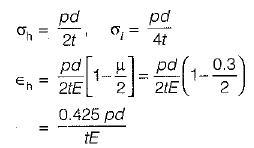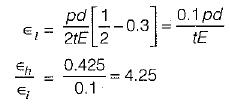QUESTION: 3

### For the same internal diameter, wall thickness, material and internal pressure, the ratio of maximum stress, induced in. a thin cylindrical and in a thin spherical pressure vessel will be

Solution: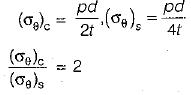QUESTION: 4

A thin cylinder contains fluid at a pressure of 500 N/m2, the internal diameter of the shell is 0.6 m and the tensile stress in the material is to be limited to 9000 N/m2. The shell must have a minimum wall thickness of nearly

Solution:

σ = pd/(2t)
t = 500 x 0.6/(2 x 9000)
= 16.57 mm = 17 mm

QUESTION: 5

In a thick-cylinder pressurized from inside, the hoop stress is maximum at

Solution:
QUESTION: 6

A thick-walled hollow cylinder having outside and inside radii of 90 mm and 40 mm respectively is subjected to an external pressure of 800 MN/m2. The maximum circumferential stress in the cylinder will occur at a radius of

Solution:

The maximum circumferential stress in a thick cylinder occurs at the inner radius, i.e. 40 mm.

QUESTION: 7

When a thin cylinder of diameter ‘d’ and thickness ‘t' is pressurized with an internal pressure of ‘p’ (1/m is the Poisson’s ratio and E is the modulus of elasticity), then

Solution:

n = [pd/(2tE)] [1-1/(2m)]
l = [pd/(2tE)[1/2 - 1/m]
t/∈n = (m - 2)/(2m - 1)

QUESTION: 8

A thin walled cyiihdrical vessel of wall thickness t and diameter d is filled with gas to a gauge pressure of p.'The maximum shear stress on the vessel wall will then be

Solution: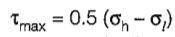= 0.5(pd/2t - pd/4t) = pd/8t

QUESTION: 9

A thin cylinder; with both ends closed is subjected to internal pressure p. The longitudinal stress at the surface has been calculated as a0. Maximum shepr ,stress at the surface will be equal to

Solution: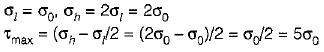QUESTION: 10

If a thick cylindrical shell is subjected to internal pressure, then hoop stress, radial stress and longitudinal stress at a point in the thickness will be -'mV

Solution: